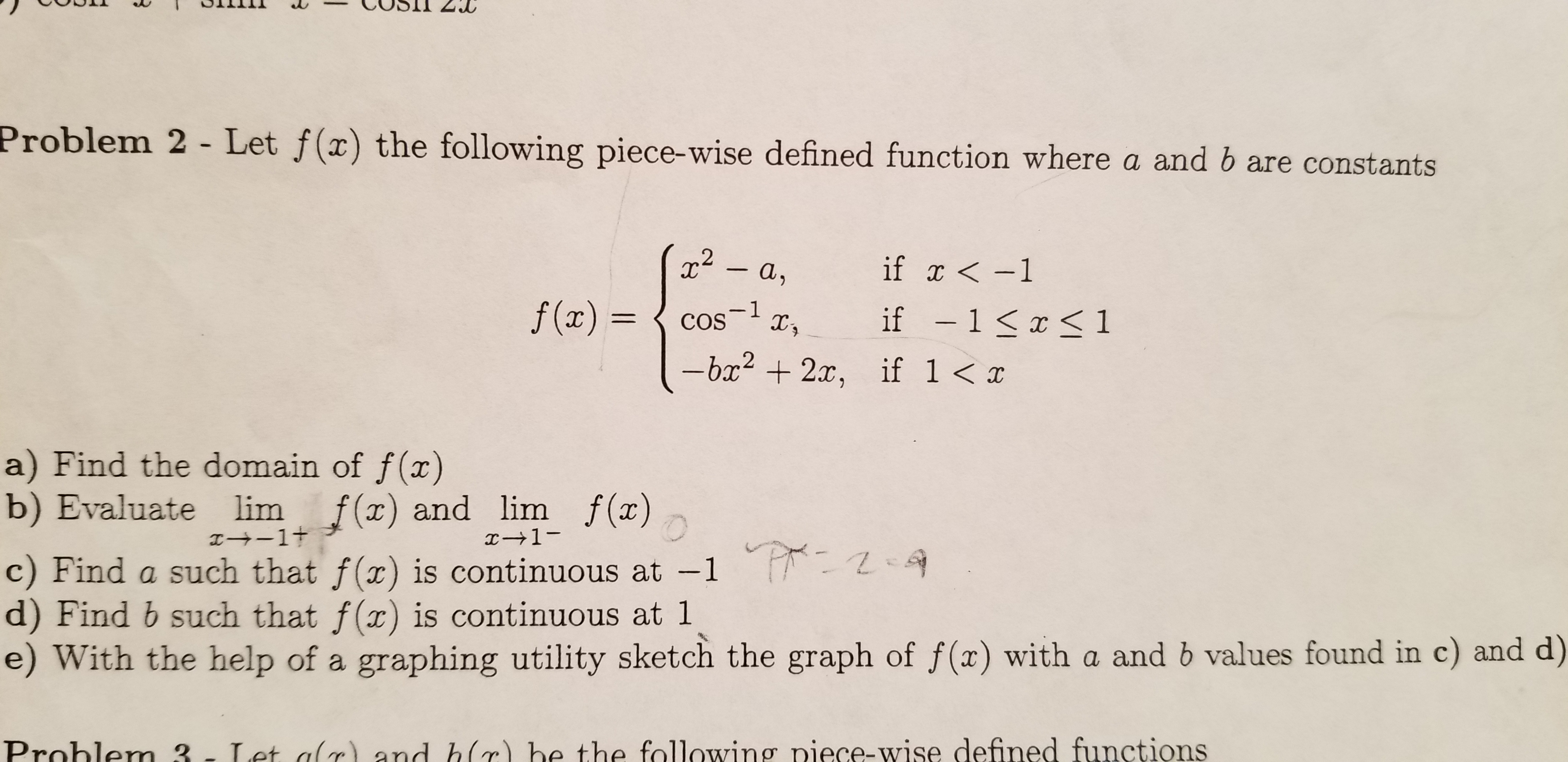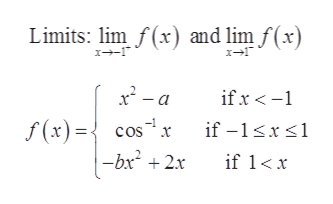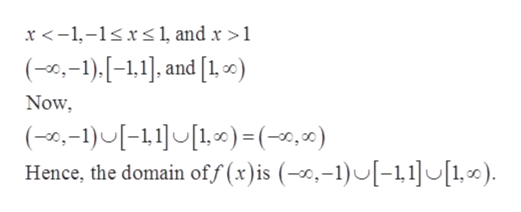Problem 2 - Let f(x) the following piece-wise defined function where a and b are constantsif x-1-a,f (x)=1COSif -1 1-bx2x,if 1a) Find the domain of f(x)b) Evaluate limI+1tf() and lim f (x)1-c) Find a such that f(x) is continuous at -1 -d) Find b such that f(x) is continuous at 1e) With the help of a graphing utility sketch the graph of f(x) with a and b values found in c) and d)Let alr and hc) be the following piece-wise defined functionsProhlem 3-

Question

Problem 2 in attached imagehelp_outlineImage TranscriptioncloseProblem 2 - Let f(x) the following piece-wise defined function where a and b are constants if x-1 -a, f (x)= 1 COS if -1 1 -bx2x, if 1 a) Find the domain of f(x) b) Evaluate lim I+1t f() and lim f (x) 1- c) Find a such that f(x) is continuous at -1 - d) Find b such that f(x) is continuous at 1 e) With the help of a graphing utility sketch the graph of f(x) with a and b values found in c) and d) Let alr and hc) be the following piece-wise defined functions Prohlem 3- fullscreen
Step 1

Note: Since we only answer up to 3 sub-parts, we’ll answer the first 3.

Please resubmit the question and specify the other subparts (up to 3) you’d like answered.

Provided that the function f(x) is piece wise defined function where a and b are constants it is required to determine the following:

Part a) The domain of f(x).

Part b) The limit of the function given below:

Part c) The value of a for which f(x) is continuous at -1.help_outlineImage TranscriptioncloseLimits: lim f(x) and lim f(x) x2-a ifx- f(x) ={ cos -bx 2x -1 if -1x if 1x fullscreen
Step 2

The domain of the function is the set of all possible values of x for which the function is defined.

The function f(x) is continuous at a point a is the right-hand limit, the left-hand limit of the function and value of the function at the point a exists and are equal.

Step 3

Part a)

The piece wise function is defined...help_outlineImage Transcriptionclosex <-1,-1sxs 1, and x >1 (--1),[-1,1]. and [1,0) Now (0,-1)-111.0) =(-0,0) Hence, the domain off (x)is (,-1)[-1,11,0) fullscreen

Want to see the full answer?

See Solution

Want to see this answer and more?

Our solutions are written by experts, many with advanced degrees, and available 24/7

See Solution
Tagged in

Calculus